[BACK]
 Computers, Materials & ContinuaDOI:10.32604/cmc.2022.019347Article

Optimal Tuning of FOPID-Like Fuzzy Controller for High-Performance Fractional-Order Systems

1College of Engineering at Wadi Addawaser, Prince Sattam Bin Abdulaziz University, Al-Kharj, 11911, Saudi Arabia
2Department of Computers and Automatic Control Engineering, Faculty of Engineering, Tanta University, Tanta, 31733, Egypt
3Department of Electrical Engineering, Faculty of Engineering, Minia University, Minia, 61517, Egypt
*Corresponding Author: Ahmed M. Nassef. Email: a.nasef@psau.edu.sa
Received: 10 April 2021; Accepted: 14 May 2021

Abstract: This paper addresses improvements in fractional order (FO) system performance. Although the classical proportional–integral–derivative (PID)-like fuzzy controller can provide adequate results for both transient and steady-state responses in both linear and nonlinear systems, the FOPID fuzzy controller has been proven to provide better results. This high performance was obtained thanks to the combinative benefits of FO and fuzzy-logic techniques. This paper describes how the optimal gains and FO parameters of the FOPID controller were obtained by the use of a modern optimizer, social spider optimization, in order to improve the response of fractional dynamical systems. This group of systems had usually produced multimodal error surfaces/functions that occasionally had many variant local minima. The integral time of absolute error (ITAE) used in this study was the error function. The results showed that the strategy adopted produced superior performance regarding the lowest ITAE value. It reached a value of 88.22 while the best value obtained in previous work was 98.87. A further comparison between the current work and previous studies concerning transient-analysis factors of the model’s response showed that the strategy proposed was the only one that was able to produce fast rise time, low-percentage overshoot, and very small steady-state error. However, the other strategies were good for one factor, but not for the others.

Keywords: Fuzzy-logic control; fractional-order systems; parameter estimation; optimization

1  Introduction

Fuzzy-logic control (FLC), established by the use of integer orders, has been used in several engineering applications, including photovoltaic , fuel-cell , thermoelectric generation , and biodiesel  systems. In engineering applications, numerous dynamic systems can be controlled more precisely by the use of fractional orders (FOs). However, recently the use of FLC systems with FOs instead of integer orders has produced a significant improvement in system performance . FLC systems provide an alternative approach to design controllers by using empirical data. These data are generated from a human operator who directly controls the procedure. The operator identifies a set of rules for controlling the process. These rules are then included in the fuzzy controller to mimic the decision-making procedure of the operator. Fractional FLC is the result of integrating the traditional FLC and FO techniques. This integration exhibits improved performance for a wide range of dynamic systems compared to conventional FLC.

Control systems based on fractional calculus have recently been drawing growing attention in research, due to their extra flexibility and improved design performance . Fractional calculus has been used in such engineering applications as signal processing, process control , nuclear reactor control , and chaos synchronization . Fractional calculus has also been integrated into different controllers to improve their performance. The FO proportional–integral–derivative (FOPID) controller is a generalized controller of conventional PID. It offers superior response and more stability compared to conventional PID  yet determining the parameters of FOPID is a dilemma. Five parameters need to be identified, instead of only three in the case of conventional PID. Consequently, several tuning approaches have recently been established to determine the parameters of FOPID .

FOPID has been demonstrated to be an effective controller in several complex nonlinear systems . Li et al.  designed a fuzzy-PID controller for a nonlinear hydraulic turbine–governing system. Mohanty et al.  conducted an analytical study of a FOPID controller with a derivative filter for automatic generation control in a multiarea power system. Xu et al.  studied an adaptive controller based on FLC for a pumped storage unit. Arya et al.  proposed a FOPID controller for automatic generation control of multizone, multisource power-generation systems. These studies have proven that the FOPID controller shows better performance and robustness.

The current research aimed to determine the optimal gains and FO parameters of FOPID when using social spider optimization (SSO) to improve the response of fractional dynamic systems. This type of system usually produces a multimodal error surface that occasionally has many local minima. During the optimization process, the gains and FO parameters of PID are used as the decision variables, whereas the integral time of the absolute error (ITAE) is assigned as the objective function. The results are compared with genetic algorithms (GAs), particle-swarm optimization (PSO), harmony search (HS), gravitational search algorithms (GSA), and cuckoo search (CS).

This paper is organized as follows. In Section 2, the concept of a FOPID-like fuzzy controller is introduced. In Section 3, a brief description of the SSO algorithm is presented. Section 4 presents the discussion of the results obtained and comparative testing. Finally, in Section 5, the main findings are outlined.

2  FOPID-Like Fuzzy Controller

In the classical PID controller, the controlling signal (control action) is calculated according to proportion of error, integral of error, and derivative of error. The constants of proportionality are the controller’s gains. They are usually named KP, KI, and KD for proportional, integral, and derivative gains, respectively. The control action and transfer function of the PID controller as a function of the system’s error are shown in Eqs. (1) and (2), respectively:

u(t)=KPe(t)+KI0te(t)dt+KDde(t)dt (1)

U(s)E(s)=KP+KIs+KDs (2)

FOPID is an example of the use of fractional calculus in control systems. The modification in the controller’s transfer function includes the derivative and integral terms by changing the Laplace complex frequency, s, to accept FOs. Therefore, FOPID becomes:

U(s)E(s)=KP+KIsλ+KDsα (3)

where λ and α are two positive real numbers.

Since the concept’s introduction in 1965, FL has become an effective technique in industrial applications. Accordingly, FL added a new perspective to the control theory with the aim of formulating the relationship between input and output variables. Previously, this relationship had been represented mathematically. However, in the sense of FL, the relationship between inputs and outputs can be represented by a set of “if–then” rules. Every rule signifies a portion in the input–output space. Therefore, signal processing in the fuzzy controller passes through the three processing operations like a normal fuzzy system. In other words, every input should be fuzzified (converted from crisp to fuzzy) through its associated fuzzy membership functions (MFs). These fuzzy inputs are passed to the rules in the knowledge base in the inference engine to produce the rules’ fuzzy outputs. The overall fuzzy output is obtained by the aggregation (union) of the fired fuzzy rules. Finally, the defuzzification (conversion from fuzzy to crisp) operation takes place to come up with the final output value. In control systems, a Mamdani-type fuzzy rule is preferable for most systems:

IF error is NS and change-of-error is PS, THEN control action is Z

where NS is negative small, PS positive small, and Z zero MFs.

Two crucial parameters have to be set properly in the design of a fuzzy controller. The first is the controller’s inputs and their associated MFs. The second is the fuzzy rule–based list. In classical PID, the control-action value is based on information about the system’s error, integration of error, and derivative of error. However, in fuzzy control, integration of error cannot produce sufficient information to take any action based on its value. Therefore, information related to the integration term can be obtained by considering the controller’s input as a derivative term, then integrating the controller’s output. In this respect, two configurations can be adopted to implement a PID-like fuzzy controller. The first is to build both a PD and a PI fuzzy controller, and sum their outputs. The following is an example of two fuzzy rules for PD and PI controllers, respectively:

IF error is NS and change in error PS, THEN control action is Z

IF error is NS and change in error PS, THEN change in control action is Z

The PI-like fuzzy controller of the first configuration is shown in Fig. 1.

Fortunately, the same rule base can be used for both PD and PI controllers. The second configuration of the PID-like fuzzy controller is to use a combination of PD and PI controllers, as shown in Fig. 2. It can be seen from the figure that the configuration is composed of a PD-like fuzzy controller added to a classical integrator. The latter configuration is adopted in many industrial processes.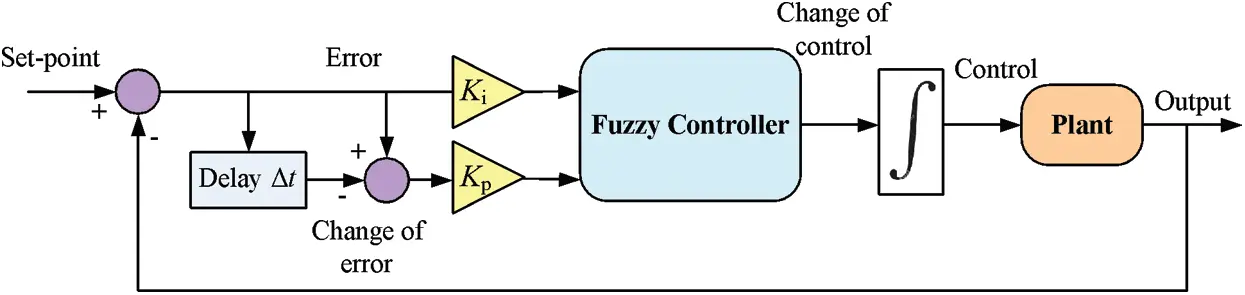Figure 1: PI-like fuzzy-controller closed-loop configuration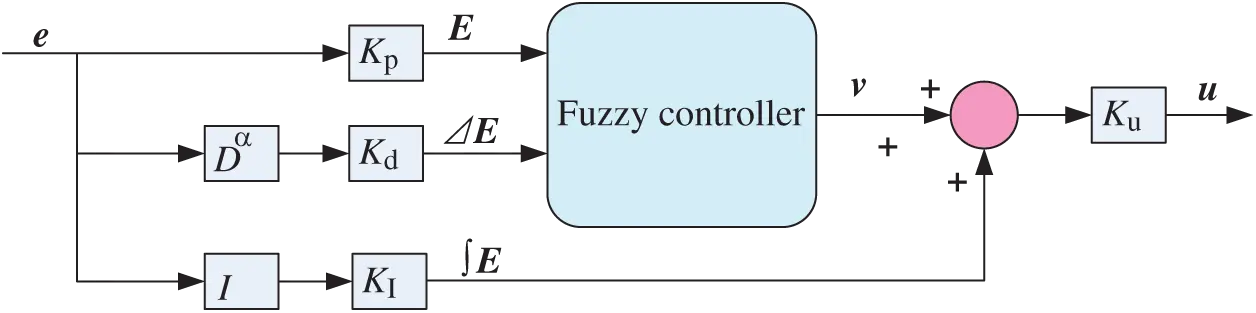Figure 2: FO PD + I fuzzy-controller configuration

In this study, the error (E) and change in error (Δ E) are considered the fuzzy controller inputs. Each input is represented by seven MFs that cover the universe of discourse in the range [−1, 1]. Usually, the rule base is built with the help of an expert who fully understands the system’s behavior and the controller’s dynamics. PD-like fuzzy controller rules are listed in Tab. 1. Linguistic variables of the MFs are N and P, denoting negative and positive, respectively, and B, M, S, and Z, denoting big, medium, small, and zero, respectively.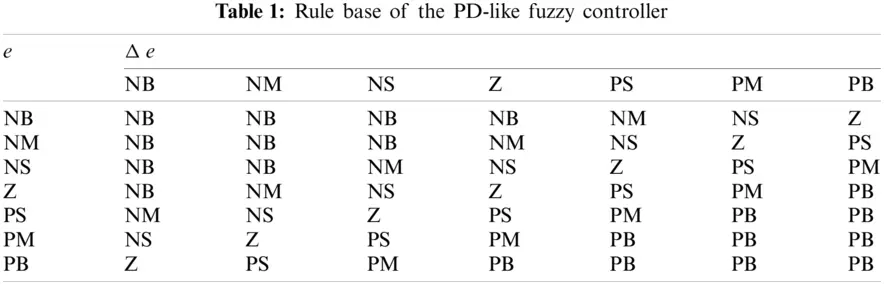3  Optimal SSO-Based Parameters

In this work, SSO, a recent and efficient optimizer, was applied to obtain optimal gains and optimal FOs for the controller. SSO simulates the cooperative behavior of spiders within a colony. It uses a population (S) of N candidate solutions, where every solution represents a spider position, whereas the general web symbolizes the search space—X. During the optimization process, every spider (i) maintains a weight (wi) based on its best solution:

wi=fitnessiworstbestworst (4)

where fitnessi denotes the value of the cost function of the ith spider’s position. An important feature of SSO is the exchange of information among spiders through the vibrations produced in the communal web. This feature is modeled thus:

Vi,j=wjedi,j2 (5)

where wj denotes the spider weight and di,j2 the distance between spiders i and j.

The update process depends on the sex. For the female, these equations can be used:

fik+1={fik+α.Vi,c.(Scfik)+β.Vi,b.(Sbfik)+δ.(r12)probability(PF)fikα.Vi,c.(Scfik)β.Vi,b.(Sbfik)+δ.(r12)probability(1PF) (6)

where α, β, δ, and r denote random values, K the iteration number, Vi, c the vibration transferred by the closest individual (c), and Vi, b the vibration transferred by the best solution. For male members, this update equation can be used:

mik+1={mik+α.Vi,f.(Sfmik)+δ.(r12)ifmikDmik+α.(hNDmhk.whhNDwh)ifmikND (7)

where Sf represents the nearest female element to the individual male and Vi, f the vibration transferred by the nearest female spider. More details about the algorithm’s mathematical modeling and physical illustration can be found in Cuevas et al. .

The objective function is used as in Eq. (3), with a time range (T) of 3 s. The configuration of the optimization process is shown in Fig. 3.

To optimally track the system’s input, the tracking error should be as minimal as possible. Accordingly, the performance of a controller is best evaluated in terms of the error criterion. Most optimization techniques use the objective function in terms of system error. However, the most popular performance-assessment criteria are integral of absolute errors, integral square of errors, mean-squared error, and ITAE. In this work, ITAE was selected as the objective function  for a fair comparison with the results obtained by Cuevas et al. . The formulae for the objective function are:

J(x)=0Tte(t)dt (8)

e(t)=r(t)y(t) (9)

where e(t), r(t), and y(t) are system error, unit-step reference value, and system output, respectively, T system-response time, and x controlling-variable vector containing the controller gains KP, KI, KD, and KU, as well as the controller FOs—λ and α.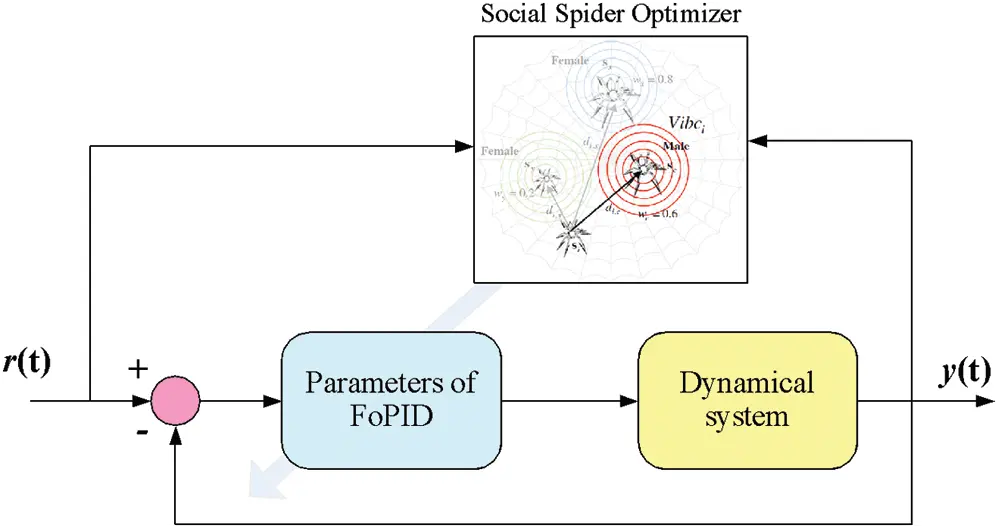Figure 3: FOPID-like fuzzy controller–optimization process

Systems’ performances are usually compared by their behavior in the transient-response phase. This can be done using quantitative markers. The most important markers of a system’s response include rise time (tr), delay time (td), steady-state time (ts), peak time (tp), percentage overshoot (%OS), and steady-state error (Ess). Fig. 4 shows the step response of the second-order system on the main performance indices. In this work, we adopted the most important markers: tr, %OS, and Ess.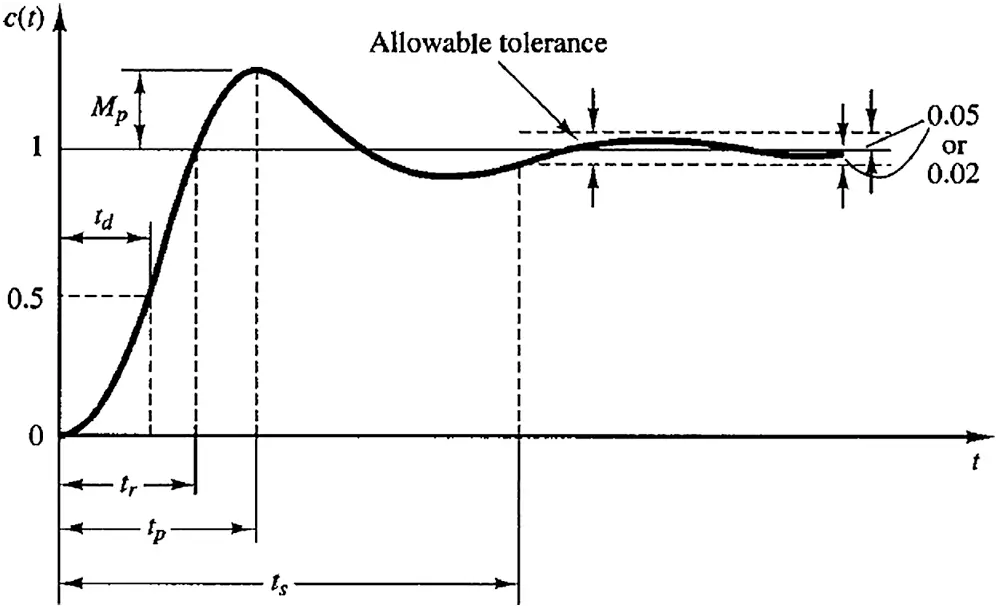Figure 4: Transient-response specifications of second-order system

tr defines the time elapsed for the system’s output to go from 10% to 90% of its final value and %OS is the percentage of the difference between output and input at the first peak, Mp, over output at the steady state—y(∞):

%OS =Mpy()y()100 (10)

Ess is the difference between the reference value and the final value of the system’s response. For a unit-step response, it can be calculated as Ess = 1 – y(∞), where y(∞) is the steady-state value of the system’s response.

4  Results and Discussion

The closed-loop system, comprising the FO system as the controlled process and the FOPID fuzzy controller, was implemented using MatLab R2020b and its Simulink toolbox. The FO part was implemented using the Fomcon toolbox. In this work, seven triangular MFs were selected as the fuzzification functions for the inputs and the output, which resulted in 49 Mamdani-type fuzzy rules. The output was defuzzified using center-of-gravity defuzzification method.

In this study, the system under consideration included a fractional dynamical system that usually produces a multimodal error surface with many local minima. The transfer function of the fractional system is presented in Eq. (11):

G=1s1.5+1 (11)

The configuration of the closed-loop model of the proposed FOPID fuzzy controller that controls the fractional system is presented in Fig. 5.Figure 5: The closed-loop model of the proposed FOPID fuzzy controller

The results of the strategy proposed compared to previous work in the literature are presented in Tab. 2. The table shows that adding a fractional integrator produced better performance with regard to lowest ITAE value compared to other strategies.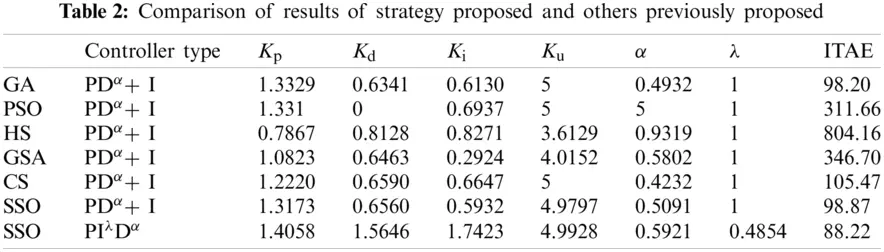Fig. 6 presents plots of the system’s response at the resulting optimal gains and FOs obtained through the technique proposed, as well as responses obtained with previous techniques.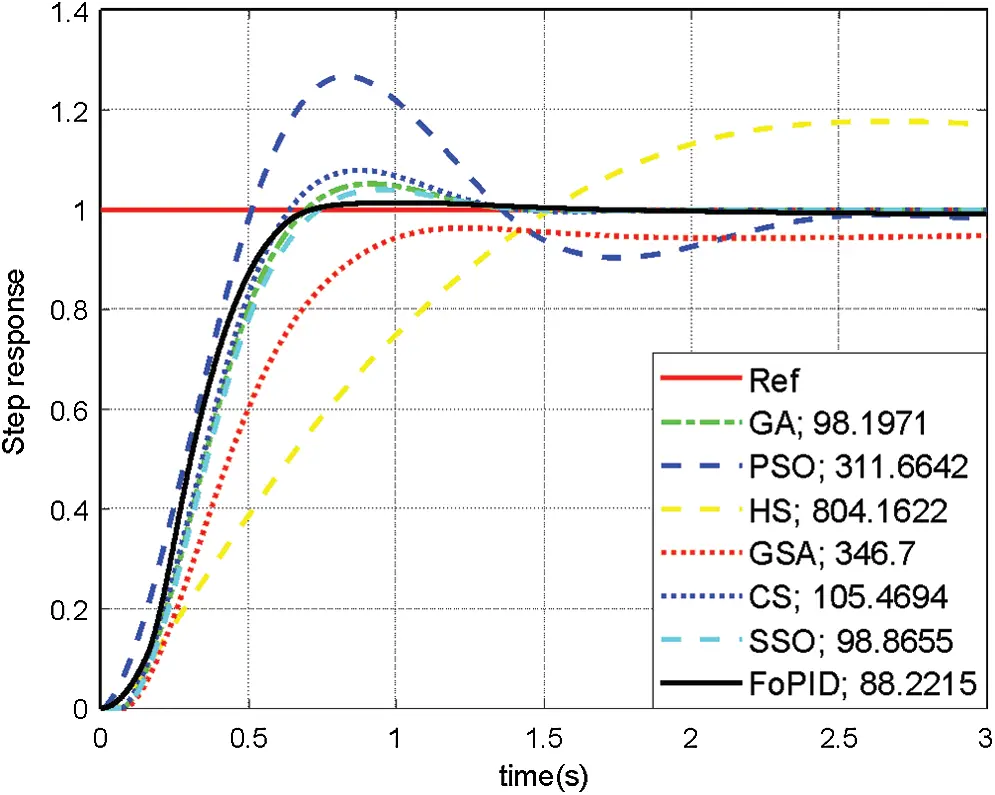Figure 6: Step response of the FOPID fuzzy controller vs. previous strategies

The comparison was extended to measure transient-response factors. This analysis included calculation of system tr, %OS, and Ess. The resulting values of all factors for the strategy proposed in comparison to the other strategies are shown in Tab. 3. FOPID provided the best set of transient-response factors. It yielded fast tr, low %OS, and small Ess, the only strategy to accomplish this. The findings of this study confirm that the combination of FL and fractional calculus can provide a robust and efficient FOPID-like fuzzy controller that can improve the performance of complex systems, such as FO ones.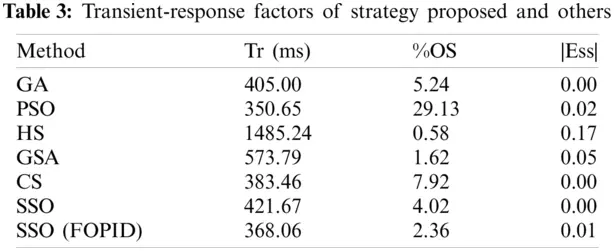5  Conclusion

This study describes the use of a FOPID-like fuzzy controller to enhance the performance of FO systems. The properly set values of the controller played a vital role in the system’s performance. The optimal parameters of the controller relative to its gains and the FOs were obtained using SSO. The resulting gains and FOs produced the best (lowest) ITAE value: that obtained using the strategy proposed exceeded the previous best in the literature by 10.77%. Transient-response factors showed better performance in terms of fast tr, low %OS, and small Ess. Consequently, the findings of this study prove that compared to other controllers, the use of a FOPID-like fuzzy controller can produce outstanding performance in linear or nonlinear systems.

Funding Statement: The authors received no specific funding for this study.

Conflicts of Interest: The authors declare that they have no conflicts of interest to report regarding the present study.

## References

1. M. Aly and H. Rezk, “A differential evolution-based optimized fuzzy logic MPPT method for enhancing the maximum power extraction of proton exchange membrane fuel cells,” IEEE Access, vol. 8, pp. 172219–172232, 2020.
2. W. H. Tanveer, H. Rezk, A. M. Nassef, M. A. Abdelkareem, B. Kolosz et al., “Improving fuel cell performance via optimal parameters identification through fuzzy logic based-modeling and optimization,” Energy, vol. 204, pp. 117976, 2020.
3. N. Kanagaraj, H. Rezk and M. R. Gomaa, “A variable fractional order fuzzy logic control based MPPT technique for improving energy conversion efficiency of thermoelectric power generator,” Energies, vol. 13, no. 17, pp. 4531, 2020.
4. A. M. Nassef, E. T. Sayed, H. Rezk, M. A. Abdelkareem, C. Rodriguez et al., “Fuzzy-modeling with particle swarm optimization for enhancing the production of biodiesel from microalga,” Energy Sources, Part A: Recovery, Utilization, and Environmental Effects, vol. 41, no. 17, pp. 2094–2103, 2019.
5. E. Cuevas, A. Luque, D. Zaldívar and M. Pérez-Cisneros, “Evolutionary calibration of fractional fuzzy controllers,” Applied Intelligence, vol. 47, no. 2, pp. 291–303, 2017.
6. I. Pan and S. Das, “Fractional order fuzzy control of hybrid power system with renewable generation using chaotic PSO,” ISA Transactions, vol. 62, pp. 19–29, 201
7. S. Das, I. Pan and S. Das, “Performance comparison of optimal fractional order hybrid fuzzy PID controllers for handling oscillatory fractional order processes with dead time,” ISA Transactions, vol. 52, no. 4, pp. 550–566, 2013.
8. S. Das, I. Pan and S. Das, “Fractional order fuzzy control of nuclear reactor power with thermal-hydraulic effects in the presence of random network induced delay and sensor noise having long range dependence,” Energy Conversion and Management, vol. 68, pp. 200–218, 2013.
9. S. Das, I. Pan, S. Das and A. Gupta, “Master-slave chaos synchronization via optimal fractional order PI λ D μ controller with bacterial foraging algorithm,” Nonlinear Dynamics, vol. 69, no. 4, pp. 2193–2206, 2012.
10. R. Pradhan, S. K. Majhi, J. K. Pradhan and B. B. Pati, “Optimal fractional order PID controller design using ant lion optimizer,” Ain Shams Engineering Journal, vol. 11, no. 2, pp. 281–291, 2020.
11. X. Wu, Y. Xu, J. Liu, C. Lv, J. Zhou et al., “Characteristics analysis and fuzzy fractional-order PID parameter optimization for primary frequency modulation of a pumped storage unit based on a multi-objective gravitational search algorithm,” Energies, vol. 13, no. 1, pp. 137, 2020.
12. C. Li, Y. Mao, J. Zhou, N. Zhang and X. An, “Design of a fuzzy-PID controller for a nonlinear hydraulic turbine governing system by using a novel gravitational search algorithm based on cauchy mutation and mass weighting,” Applied Soft Computing, vol. 52, no. 13, pp. 290–305, 2017.
13. P. K. Mohanty, B. K. Sahu, T. K. Pati, S. Panda and S. K. Kar, “Design and analysis of fuzzy PID controller with derivative filter for AGC in multi-area interconnected power system,” IET Generation, Transmission & Distribution, vol. 10, no. 15, pp. 3764–3776, 2016.
14. Y. Xu, Y. Zheng, Y. Du, W. Yang, X. Peng et al., “Adaptive condition predictive-fuzzy PID optimal control of start-up process for pumped storage unit at low head area,” Energy Conversion and Management, vol. 177, no. 9, pp. 592–604, 2018.
15. Y. Arya and N. Kumar, “BFOA-scaled fractional order fuzzy PID controller applied to AGC of multi-area multi-source electric power generating systems,” Swarm and Evolutionary Computation, vol. 32, no. 1, pp. 202–218, 2017.
16. E. Cuevas, M. Cienfuegos, D. ZaldíVar and M. Pérez-Cisneros, “A swarm optimization algorithm inspired in the behavior of the social-spider,” Expert Systems with Applications, vol. 40, no. 16, pp. 6374–6384, 2013.This work is licensed under a Creative Commons Attribution 4.0 International License, which permits unrestricted use, distribution, and reproduction in any medium, provided the original work is properly cited.